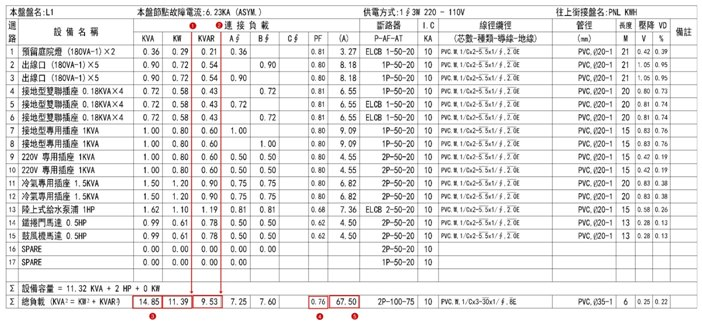## 總負載

2016/8/16 下午 10:20視在功率S（KVA） 有效功率P（KW） 無效功率Q（KVAR） 總負載 ΣS = √（ ΣP² + ΣQ² ） ΣP = P1 + P2 + … ΣQ = Q1 + Q2 + … 需量 DF = 0.8 ΣS * 0.8 ΣP * 0.8 ΣQ * 0.8 功率因數改善 ΣS = √（ （ΣP * 0.8）² + ΣQ’² ） ΣP * 0.8 ΣQ’ =ΣQ * 0.8 - QscΣ P = 0.29 + 0.72 * 2 + 0.58 * 3 + 0.8 * 4 + 1.2 * 2 + 1.1 + 0.61 * 2 = 11.39 KW

Σ Q = 0.21 + 0.54 * 2 + 0.43 * 3 + 0.6 * 4 + 0.9 * 2 + 1.19 + 0.78 * 2 = 9.53 KVAR

Σ S = √( Σ P² +Σ Q² ) = √(11.39² + 9.53² ) = √(220.553) = 14.85 KVA